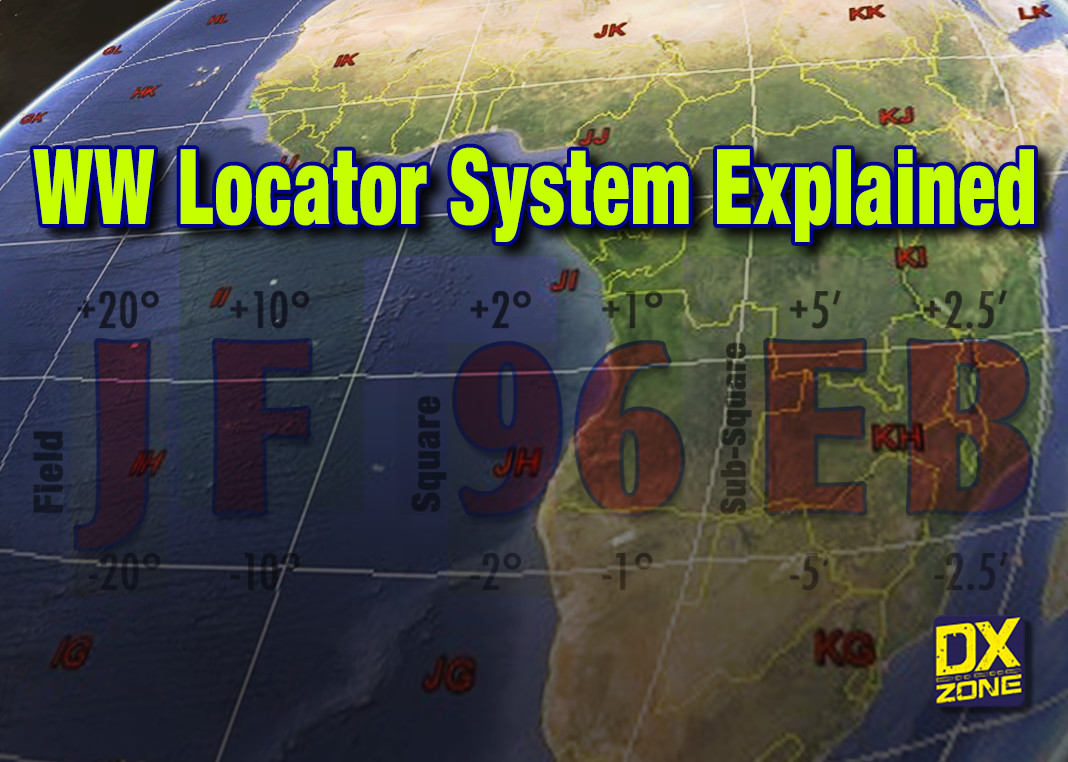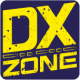# Grid Square Locator System

How the Grid Square Locator System Works and its origins

25000The Grid Square Locator, also known as the Maidenhead Locator System, is a geographical co-ordinate method based on a 6-digit code, widely used by amateur radio operators to determine a rough position on the Earth.

The grid locator system is one of the many peculiarities that characterize the amateur radio world, and of which all radio amateurs are or should be aware.

Not all radio amateurs, and especially the youngest ones, however, do not know the history of the birth of this clever, although crude, method of calculating position on earth.

QTH Locator, QRA Locator, Maidenhead Locator and WW Grid Locator system are all different terms, often used to identify the same system, that should sound familiar to you. Here we will try to recap the history and the origin of all these names.

## History of the Grid Locator System

The current Grid Square Locator System, as we know it today, is the evolution of a previous method, invented in the late 1950s with the goal to help in calculating scores for VHF contests. This system has been in use by European hams for some decades,

### The QRA Locator

To facilitate the measurement of this distance, DL3NQ introduced at the DL VHF meeting in Weinheim on 1958, a method named QRA-Kennen based on a five-digits code.

The system used two letters to indicate the largest unit, “Square”, that was 2 degrees longitude * 1 degree latitude. Without repetitions the system covered the area 0-52 degrees Eastern longitude and 40-66 degrees Northern latitude.

The QRA-Locator consisted of a five-character code with two capital letters, a two-digit number and a lower-case letter, for example AB55m.

This new system was successfully tested in the Autumn of 1958, during OK VHF contests, and after positive results, during The Hague VHF meeting in October 1959 it was decided to submit this method named, QRA-Locator as official IARU R1 recommendation.

### The QTH Locator

At the Region 1 Conference in Opatija in 1966 this map was adopted as the official Region 1 QRA-Locator map, while the name changed to “QTH Locator” later in 1972.

When amateurs outside Region I, especially in North America (Region II), became interested in using this system they found it ambiguous, and not applicable elsewhere because squares were not unique, and same zone could be repeated in several zones of the Earth.

It soon became obvious that for other regions to adopt a system similar to the European one, a different algorithm would have to be developed.

A new global method was discussed and proposed, it was based on a six-digits codes determined by an elaboration of longitude and latitudes position.

In April 1980 a meeting of European VHF managers was held in Maidenhead near London, UK and later in 1982 the new “Maidenhead Locator System” as we know it today, was adopted by IARU Region 1 as the new locator system starting January 1, 1985.

## How the Grid Square Locator System Works

The Maidenhead Locator System permits to represent a position on the Earth, with just a Six-Digits code composed by letters and numbers, like JF96EB.

The Locator system is based on three elements, Fields, Squares and Sub-Squares, having different sizes, and a rectangular shape (not properly a square) where each element is represented by a pair of digits.

### Elements of the Grid Square System

• The World is first divided into (18 * 18) 324 very large areas named Fields.
• Each Field is divided by 100 Squares
• Each Square is divided into 576 Sub-Squares

## Grid Square Maps

Here below you can find two interesting Maidenhead locator maps in PDF file that can be easily scaled, zoomed and printed even in large format.

## The Grid Square code

Not everyone knows how the code is composed and how it works. Each digit of the code has its own specific meaning and corresponds to a specific coordinate value, be it a longitude or a latitude.

On a full grid locator like JF96EB, three characters (first, third and fifth E 7 D) specify the longitude, the remaining three characters (M 7 A) specify latitude.

The first character is the longitude and the second character is the latitude on each level. The numbering is always West to East and North to South.

The first two characters E and M, represents the FIELD, third and fourth numbers represent the SQUARE, while the fifth and sixth caharcters represent the sub-square.

Now, we try to understand possible values each element can assume, and which are increments in latitude and longitude.

### The Fields

• The World is first divided into (18 * 18) 324 very large areas named Fields.
• Fields measure 20 degrees longitude * 10 degrees latitude.
• Fields are indicated by two letters AA – RR

The First character (E), that is always a letter of a grid locator, specifies a longitude in increments of 20°, and it can vary its value from the letter A corresponding to the interval 180°W to 160°W, (the letter B correspond to the interval 160° W to 140°W and so on) till the letter R, which corresponds to 160° E to 180° E longitude.

The Second character in a grid locator specifies the latitude in 10° increments.For instance, the letter A covers from the interval from 90°S to 80° S, B covers from 80°S to 70°S and so on until R, which specifies latitudes from 80° N to 90 N.

A to R is therefore the possible range of letters for the first two characters.

West Longitudes and South Latitudes are treated as negative values in the grid locator system, while east longitudes and north latitudes are positive.

### The Squares

• Each Field is divided by 100 Squares
• Squares : 2 degrees longitude * 1 degree latitude.
• Squares by two digits 00 – 99

Third and fourth characters are digits in the range 0 to 9. The third character divides longitudes lines into 2° increments. The fourth character divides latitude zones into 1° increments.

### The Sub-Squares

• Each Square is divided into 576 Sub-Squares
• Sub-squares by two letters AA – XX.
• Sub-squares : 5 minutes longitude * 2,5 minutes latitude.

The last two characters are always Letters in a grid locator, subdivide the 2° x 1° rectangles designated by arc in size.
The letter A through X corresponds to each coordinate  of these 5’ x 2.5’ rectangles.

A to X is therefore the possible range of letters for the last two characters.

We will learn below how to calculate the grid square starting from latitude and longitude coordinates, but nowadays if you have an internet connection is very easy to locate a position of the world and determine the exact locator just with a point and click.

Here below a selection of some interesting resources available online

## How to calculate the Grid Square Locator

Here we want to try to demonstrate how to determine the classic six-digit grid square locator, converting latitude and longitude coordinates into the WW QTH Locator.

Calculations will determine at first the Field, the Square and the Sub-Square.

In this calculation, we will need to determine the integer digit corresponding to each value, and it is important to consider the remainder of each division, used in the subsequent calculation.

Let’s take an example, we need to determine the Grid Squale Locator of Signal Hill in Cape Town South Africa, and from google maps we learn that is located at:

-33.934562 Latitude / 18.388544 Longitude

### Field Calculation

The world is divided into a 18×18 fields, measuring 20°x10°.
The first operation is to add to longitude, 180° in order to work with positive numbers, since we need to determine a non-negative number. Afterward we will divide the reuslt by 20 (degrees) and we will consider the integer number obtained and its remainder.
The letter, will correspond to the obtained number. Same calculation but adding 90 (degrees) and dividing by 10 times for Latitude.

``` A B C D E F G H I J K  L  M  N  O  P  Q  R
0 1 2 3 4 5 6 7 8 9 10 11 12 13 14 15 16 17 ```

Longitude: 18.388544 degrees
= 18.388 + 180
= 198.388
=198.388 / 20
= 9 + remainder 18.388
= J (location in 10th zone)

Latitude : -33.931833 degrees
= -33.931833 + 90
= 56.068167
= 56.068167 / 10
= 5 remainder 6.068167
= F (location in 6th zone)

Resulting Field is: JF

### Square Calculation

We know that each Field is divided into 10×10 squares, measuring 2° x 1° each.
We will consider the remainders of previous Field calculation.

Longitude = 18.388
= 18.388 / 2
= 9 remainder (0.388)
= 9 (location in 9th zone)

Latitude = 6.068167
= 6.068167 / 1
= 6 remainder (0.68167)
= 6 location (location in 6th zone)

Resulting Square is: 96

### Sub-Square calculation

Each sub-square is divided into 24×24 sub-squares, measuring 5 minutes (0.083333 degrees) x2.5 minute (0.0416665 degrees).

The letter, will be the corresponding number.

``` A B C D E F G H I J K  L  M  N  O  P  Q  R  S  T  U  V  W  X
0 1 2 3 4 5 6 7 8 9 10 11 12 13 14 15 16 17 18 19 20 21 22 23 ```

Longitude = 0.388
= 0.388 : 0.083333
= 4 remainder 0,097
= E (location in 5th zone)

Latitude = 0.68167
= 0.68167 / 0.0416
= 1 remainder 0.638
= B (location in 2nd zone)

Resulting Sub-Square: EB

#### References

Additional information about history of Grid Locator system, can be found here:

Previous articleTop Amateur Radio Websites – Issue 2006
Next articleTop Amateur Radio Websites – Issue 2007Simone Mannini IW5EDI is an Amateur Radio Operator licensed since 1996. He become interested in shortwave radio listening when he was very young, and soon he got into two-way radio communications and ham radio. IT system engineer, Simone is a regular DXZone contributor, he enjoys promoting amateur radio on the internet and among youngs, he enjoys playing with antenna home brewing and DXing.

1.Melissa

Hi, thank you for posting this – I happen to know that I’m in KM56, but when I try to calculate it manually based on my lon/lat, I’m doing something wrong. Longitude is 30.67, plus 180 is 210.67, divided by 20 is 10 with 10.67 remainder… so by the chart that would put me in in L rather than K, wouldn’t it? With the latitude calculation I actually ended up two letters off.

Also, do you know why Maidenhead uses longitude before latitude? Most systems that I’m aware of do it the other way around, so it’s a bit confusing. Thank you for your help!

•DXZone

Hi Melissa thanks for comment. According to the reference table in the field calculation paragraph, 10 with 10.67 remainder fall down in K area.
Letter A is from 0 to 1, and K is for all numbers from 10 to 11. We hope this helps.

2.Meliss

oooooooh I see now – I thought we were using the remainder to decide whether to round up or down. I understand now – thank you!